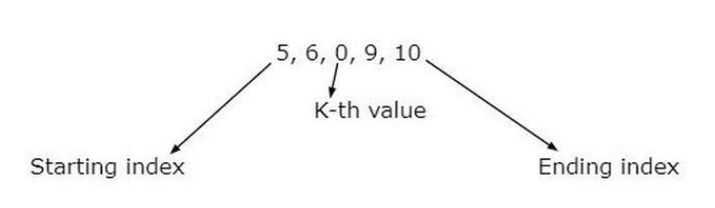# Check if it is possible to reach the index with value K when start index is given

C++ has a bitwise operator “||” to check multiple conditions at once and for finding the length of an array we use the size() function. In the given problem statement, we need to reach the K-th value which sets to 0 in the array range, and the starting index is already known. If the given index satisfies to K-th value in the array range then it will print that “We can reach the value k from the given start index”.

Let’s take an example of this −

Given integer array is 5,6,0,9,10 having a length of 4 and the start index is 2 which acts as the length of an array.So the K-th value is found at the second index of an array that verifies the possible reach to it.

In this article, we are going to check the possible reach to index k when starting index is already known.

## Syntax

vector <datatype> vector_variable_name;


## Parameters

vector − This is the keyword.

datatype − This can be any primitive data type like int, float, etc.

vector_variable name − Name provided to the vector variable

## Algorithm

• We will start the program with header files namely ‘iostream’ and ‘vector’.

• We are initializing the class name as ‘K_reach’ to define the data variables and members.

• In the private section of the class, we are initializing the ‘arr’ of type vector integer.

• In the public section of the class, we are initializing the constructor named ‘k_reach()’ where we pass the parameter as ‘inputArr’ of the type vector integer. Then storing the value of ‘inputArr’ to ‘arr’ variable that satisfies to work on the operation of K-th value.

• Again in the public section, we initialize the member function named ‘is_Reach()’ along its parameter ‘s’ and ‘k’ of integer type which will accept the value from the variables- ‘start_element’ and ‘K’ respectively(check the main function) and verifies whether it will be reached the K-th value from the start index or not.

• We are using two if-statement to return the following −

• s >= arr.size() || s < 0 || arr[s] < 0 − To find the K-th value it will check all these conditions using logical OR ‘||’ and if it is satisfied then it will return false which means the K-th value is not found.

• arr[s] == k − If both these variable matches then it will return true which means the K-th value is found.

• Then we are storing ‘arr[s]’ to integer variable ‘x’ and then set ‘arr[s]’ to ‘-1’ which will check the datatype of array elements is valid or not.

• The boolean of ‘isReach()’ function will return two conditions as follows −

• is_Reach( s+x, k) − By using this it will iterate to search the K-th index value from the beginning.

• is_Reach( s-x, k ) − By using this it will iterate to search the K-th index value from the last.

• Now we will start the main function and declare the array input.

• We are storing the value ‘4’ to the variable ‘start_element’ which acts as the length of the index position. Then store the value ‘0’ in the variable K to find the matched value from the starting index.

• We are creating the class ‘k_reach’ and taking an object named A to pass the parameter of array elements.

• We pass two parameters to the ‘is_Reach()’ function i.e. ‘start_element’ and ‘K’ which verifies the passing parameter as input.

• Finally, we are applying ‘pass’ to the if-statement to check whether the k-th value reached from starting index or not and print the result.

## Example

In this program, we are going to check the possible reach to the index with K value when the start index is given.

#include<iostream>
#include<vector>
using namespace std;
class k_reach {
private:
vector<int> arr;
public:
k_reach(vector<int> inputArr) {
arr = inputArr;
}
bool is_Reach(int s, int k) {
if ( s >= arr.size() || s < 0 || arr[s] < 0 )
{
return false;

}
if ( arr[s] == k )
{
return true;

}
int x = arr[s];
arr[s] = -1;
return is_Reach(s+x, k) || is_Reach(s-x, k);
}
};
int main() {
vector<int> arr{2,8,4,2,0,6};
int start_element = 4;

// represents as length position i.e, 0
int K = 0;
k_reach A(arr);

// here A is the object and arr is the parameter
bool pass = A.is_Reach(start_element, K);
if (pass)
cout << "We can reach the value k from the given start index.";
else
cout << "We cannot reach the value k from the given start index.";
return 0;
}


## Output

We can reach the value k from the given start index.


## Conclusion

We explored the concept of possible reach to index with a k value when the start index is given. We saw how we use the bitwise operator to give the two conditions at once and also see how the oops concept is useful to this problem statement. For example, suppose our id acts as a K value and the start index acts as a serial order of id.

Updated on: 10-May-2023

56 Views